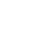# LM338 Variable Voltage Power Supply 1.25-28v, 5Amps Powerful

* LM338 Variable Power Supply *. Hello Everyone. A variable voltage power supply unit is a must for electronics, hobbyists, enthusiasts, and other people doing electronics work. A variable bench power supply unit provides a constant DC voltage output for operation and testing purposes. We can use it to power circuits with desired DC requirements and also for electronics testing circuits. So, today I am going to discuss an LM338 variable voltage power supply circuit. An LM338 is also a variable voltage regulator like LM317. But the difference is that LM338 can provide higher current outputs like 5Amp. Let’s start with our topic LM338 Variable voltage power supply.

### LM338 Voltage Regulator

LM338 is also a variable constant voltage regulator that provides the desired DC output voltage constantly. The difference between is the Output current by LM338 IC. The output power of the LM338 voltage regulator is comparatively higher than LM317 due to the higher current. The current handling capacity of this can go up to 5A and output voltage ranges from 1.25-35v dc.

The variable PSU circuit uses two output resistors along with LM338 to decide the output voltage range by the regulator. One is a fixed resistor and the other one is a potentiometer or variable resistor to set the constant desired output voltage. Below are the circuit diagram and some important factors related to how to calculate resistor values & input.

#### LM338 variable power supply 1.25- 28V DC

The above circuit is set to give max output up to 28V DC. This configuration is desired by values of R1 and R2 which is a Variable resistor or potentiometer. For example, if we want 28v then we used R1=220Ohms and R2=5K ohms. Theoretically, the calculation will be 29.6 volts but practically it will give 28v dc output. Similarly, If we want 24v max, then we will use R1=180ohms & R2= 3.3K. The values of these resistors help us to decide the maximum output voltage. According to these resistor values, we can decide the max output voltage by the power supply. Input DC voltage must be 2.3volts higher than the desired maximum output voltage. Output current is dependent on the strength of input current source.

Formula for calculating resistor values for specific voltage output is :

Vout = 1.25 * ( 1+ R2/R1)

A calculator web App is below for my users to prevent the hassle of calculating values themselves.

```  .container {
background-color: #f2f2f2;
}

```

According to these resistor values, we can decide the max output voltage by the power supply. Use this calculator to avoid the calculation hassle.

Make sure to use a good heat sink with LM338. Heat sink is required with LM338.

You can use a Load like a 24V bulb to check the intensity of the bulb increasing or decreasing according the Voltage output.

Voltage can be measured use a multimeter or digital DC voltmeter.

So, guys, I hope you people liked this tutorial and will also use this calculator. Ask me in the comments for any doubts. Also, subscribe to our newsletter for the latest post updates. Thank you.

Aabhishek
Aabhishek Sharma is a Computer Science Engineer and a Digital Marketing Trainer and Consultant. Aabhishek Sharma also loves working on Electronics Projects and working with microcontrollers and Designing Home automation Gadgets.
http://www.electroinvention.co.in

## 7 Replies to “LM338 Variable Voltage Power Supply 1.25-28v, 5Amps Powerful”

1.olabama says:

1.Aabhishek says:
2.Charles says:
1.Aabhishek says: